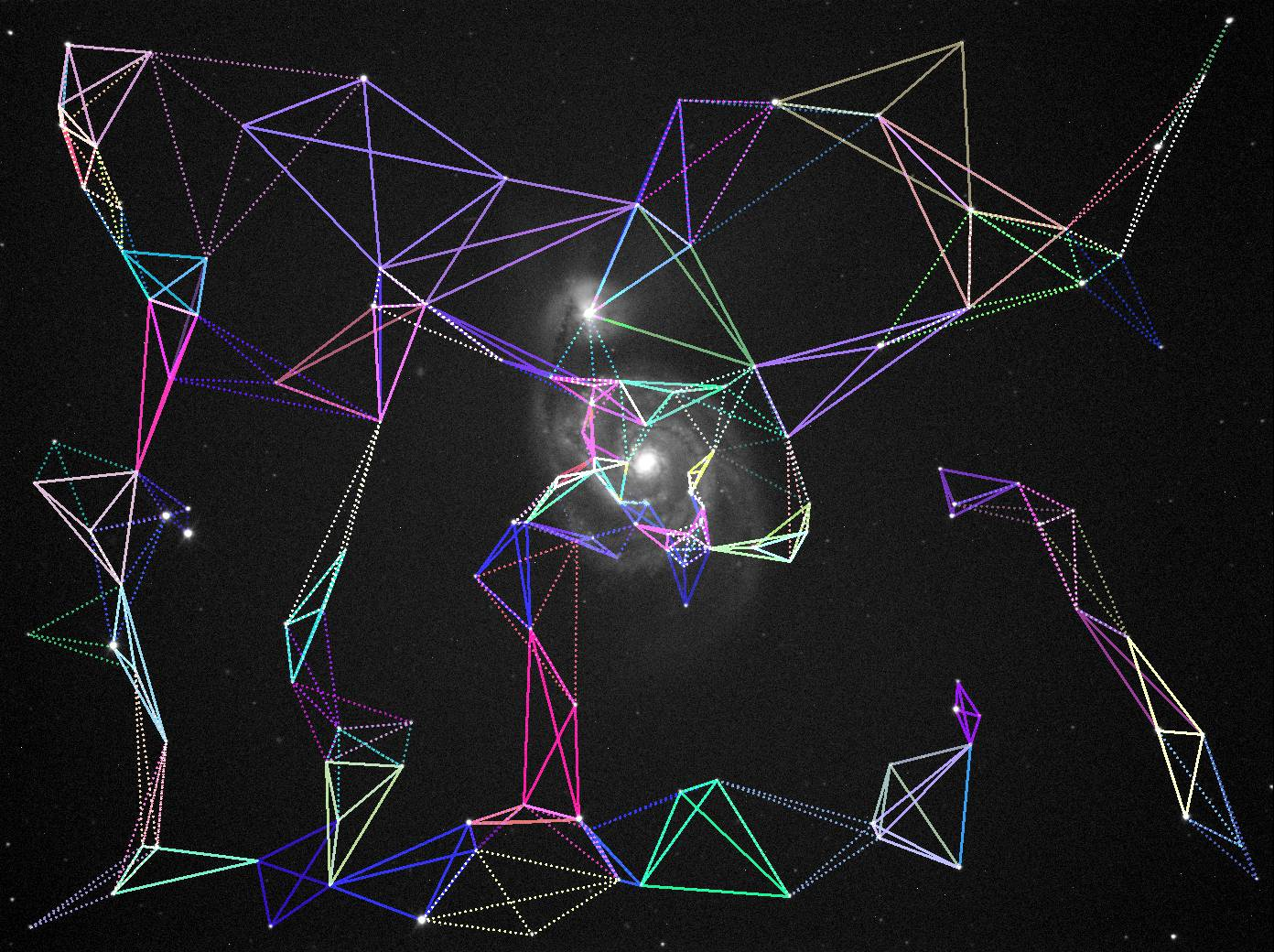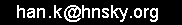www.hnsky.org

ASTAP star pattern recognition and astrometric (plate) solving algorithm.

1 Introduction to astrometric solving

1.1 How do humans do it:

Humans look for the brightest stars in the sky, determine the shortest distances between the stars (constellation lines) and estimate the angles these lines form. They see imaginary  constellation figures from close bright stars and compare them with constellations figures they have in their memory and will recognize a match.

1.2 How does the solver do it:

In ASTAP solver more or less the same process is followed. Select the brightest stars, connect them and measure the distances between them. ASTAP will search for four closest stars and measure the distances between them (the hash code or constellation). This process will be done for the image and repeated for the stars in the star database. These hash codes created from the star database will be compared with the image hash codes till some matches are found. Once some matches are found it is possible to calculate the precise position of the image with the matching database stars.

This matching process is identical as used for image aligning routine in a stack program. For aligning a match has to be found between the stars in the reference image and a second image prior to stacking. It is possible to compare the distances between two stars, three stars (triangles using the connecting lines) or four stars (quads or tetrahedrons using the connecting lines). Four stars works best with sufficient uniqueness but not being too complicated. It is not required to measure the angles.

Irregular tetrahedron figures formed by the star connection lines:1.3 So how is this done in more detail:

The image is analysed for stars and it maybe finds 2000 stars and their x, y positions. It takes the 500 brightest stars and creates from them maybe 300 tetrahedron figures of four stars. Between each four stars six lines can be drawn forming the tetrahedron. Since the image scale is specified, the distances between the four stars could be (example):

3', 2', 1', 0.7', 0.6', 0.5'

To make it independent of image size, the distance ratios are calculated by dividing the distances through the largest distance. The longest distance is kept for comparison later. So the hash code or constellation figure will consist of two parts:

Largest distance: 3'
Five ratios: 2/3, 1/3, 0.7/3, 0.6/3, 0.5/3

The five ratios are sufficient for the search. Rotation, scaling, flipping  of the image has no influence on the "five ratios".
The "largest distance" is later used to detect outliers.

From the star database numerous artificial images are created using the same star density per degree following a search pattern around the initial Ra, Dec position. The artificial images
hash codes are compared with the hash codes of the image to solve. This is the actual search. Only at about the image location a part of the hash codes will match within a small tolerance. In addition the  "largest distances" should have a fixed ratio between the image and artificial database image.

2 How does ASTAP, Astrometric STAcking Program solving works in even more detail:

ASTAP is using a linear astrometric solution for both stacking and solving.  The method is based on what traditionally is called "reducing the plate measurements". First step is to find star matches between a test image and a reference image. The reference image is either created from a star database or a reference image. The star positions x, y are to be calculated in standard coordinates which is equivalent to the sensor x,y pixel position. The x,y positions are measured relative to the image center.

The test image center, size and orientation position will be different compared with the reference image. The required conversion from the test image [x,y] star positions to the same stars on the test images can be written as:

Xref : = a*Xtest + b*Ytest + c
Yref :=  d*Xtest + e*Ytest + f

The factors, a,b,c,d,e,f are called the six plate constants and will be slightly different different for each star. They describe
the conversion of  the test image standard coordinates to the reference image standard coordinates. Using a least square routine the best solution fit can be calculated if at least three matching star positions are found since there are three unknowns.

With the solution and the equatorial center position of the reference image the test image center equatorial position, α and δ can be calculated. From the test image center using small one pixel steps in x, y, the differences in α, δ can be used to calculate the image scale and orientation.

For astrometric solving (plate solving), this "reducing the plate measurement" is done against star positions extracted from a database. The resulting absolute astrometric solution will allow specification of the α, δ equatorial positions of each pixel.
For star alignment this
"reducing the plate measurement" is done against a reference image. The result six plate constants are a relative astrometric solution. The position of the reference image is not required. Pixels of the solved image can be stacked with reference image using the six plate constants only.

To automate this process
rather then using reference stars the matching reference objects are the center positions of tetrahedrons made of four close stars.  Comparing the distance ratios between the four stars allows automated matching.

Below a brief flowchart of the ASTAP astrometric solving process:This work is licensed under a Creative Commons Attribution 4.0 International Licensewhich permits unrestricted use, distribution, and reproduction in any medium, provided the original work is properly cited.